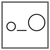Fuddlepixel coz tech is fun();

# Blog

##### Relational Operators

Let us discuss the relational operators through which we can perform relational comparison in the C programming language.

##### Arithmetic Operators

Let us discuss the arithmetic operators through which we can perform mathematical operations in the C programming language.

##### Operators Introduction

Let us get started with the operators, C has a rich set of those, to perform amazing operations with numbers and logic, making programming awesome !

Let us discuss some important concepts and awesome tricks and tips to that can be used with loops in the C the language for better coding.

##### Different kinds of loop

Let us discuss and differentiate between the different kinds of loop, namely for, while and do while available in C language.

##### Looping Repeating Concept

Let us learn the concept of looping and how to implement loops in C language, the syntax of for, while and do while loops.

Top